nextnano.com  nextnano³  Download | Search | Copyright | Publications  * password protected nextnano³ software3D hexagonal GaN QD (wurtzite)

# nextnano3 - Tutorial

## Hexagonal shaped GaN quantum dot embedded in AlN (wurtzite)

Author: Stefan Birner

If you want to obtain the input files that are used within this tutorial, please check if you can find them in the installation directory.
If you cannot find them, please submit a Support Ticket.
```-> 1DAlNGaN_BandAlignment.in -> 1DAlNGaN_BandAlignmentStrained.in -> 1DAlNGaN_BandAlignmentStrainedPzPy.in -> 1DAlNGaN_BandAlignmentStrainedPzPy_ElHl6x6.in -> 3DHexQD_GaN.in  ```

## Introduction: Conduction and valence band alignment in AlN/GaN (unstrained)

`-> 1DAlNGaN_BandAlignment.in`

The following figure shows the conduction and valence band edge alignment in AlN/GaN structures (unstrained). In AlN, the light hole (LH) is the highest valence band whereas in GaN, this is the heavy hole (HH). We assumed a valence band offset of VBO = 0.5 eV, the conduction band offset is much larger (CBO = 2.3 eV). All material parameters are based on the paper

Theory of the electronic structure of GaN/AlN hexagonal quantum dots
A.D. Andreev, E.P. O'Reilly
Phys. Rev. B 62 (23), 15851 (2000)

although meanwhile better parameters are available.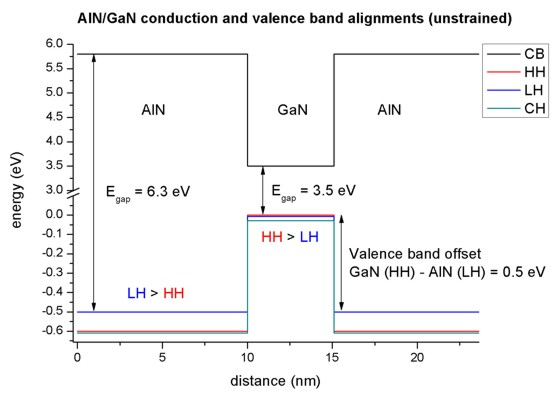## Conduction and valence band alignment in AlN/GaN of pseudomorphically strained GaN on AlN substrate

`-> 1DAlNGaN_BandAlignmentStrained.in`

The lattice constants of GaN are larger than in AlN, thus GaN is compressively strained.
The AlN band edges are the same as in the picture above, only the GaN edges have changed:

• The band gap of GaN has increased  (compressive strain increases the band gap).
• Now the crystal-field split-hole (CH) in GaN lies above the light hole (LH) and close to the heavy hole (HH).
• The valence band offset has decreased to VBO = 0.46 eV.
• The conduction band offset has decreased to CBO = 2.15 eV.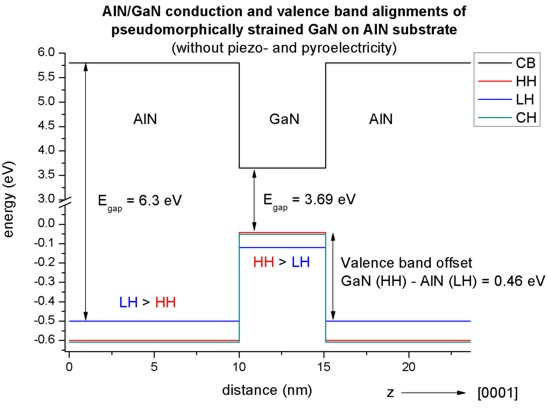## Conduction and valence band edges in AlN/GaN of pseudomorphically strained GaN on AlN substrate including piezo- and pyroelectric fields

`-> 1DAlNGaN_BandAlignmentStrainedPzPy.in`

This figure also includes the electrostatic potential which is the solution of the Poisson equation taking into account the piezo- any pyroelectric interface (and surface) charges. The piezo- and pyroelectric fields tilt the band edges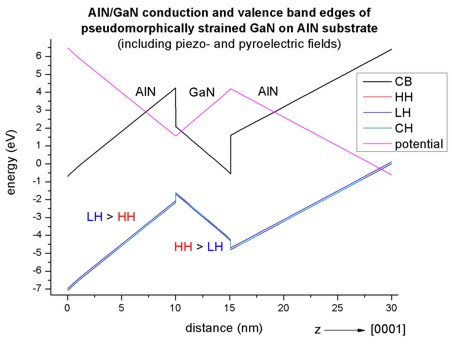## Electron and hole wave functions in an AlN/GaN/AlN quantum well

`-> 1DAlNGaN_BandAlignmentStrainedPzPy_ElHl6x6.in`

The following figure shows the electron and hole wave functions (psi²) in a 5.1 nm AlN/GaN/AlN quantum well. For the electrons, the single-band effective-mass approximation was used whereas for the holes the 6-band k.p model was used. The figure shown the four lowest electron eigenstates and the 6 highest valence band eigenstates. All eigenstates are two-fold degenerate due to spin.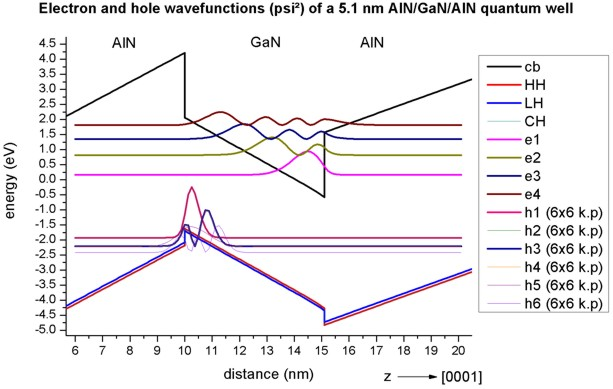## Hexagonal shaped GaN quantum dot embedded in AlN (wurtzite)

This tutorial is based on the following paper:

Theory of the electronic structure of GaN/AlN hexagonal quantum dots
A.D. Andreev, E.P. O'Reilly
Phys. Rev. B 62 (23), 15851 (2000)

The simulated hexagonal GaN quantum dot (height = 4 nm) is embedded in an AlN matrix. The wetting layer is 1 nm thick and consists of GaN.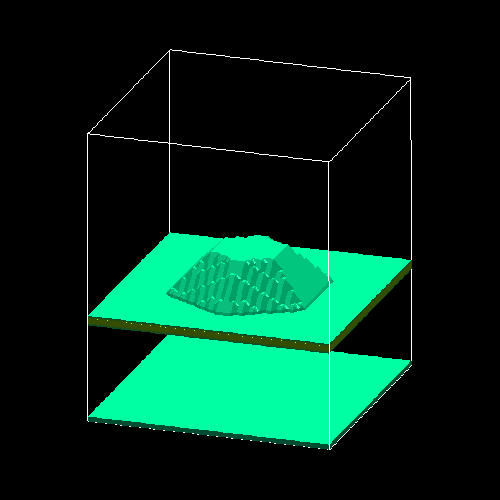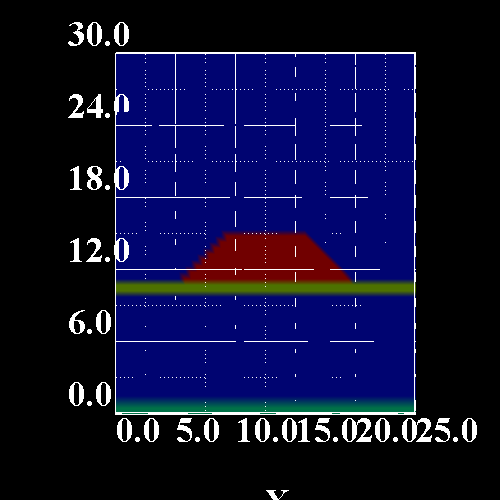The strain tensor components of a line through the center of the quantum dot along the z axis are shown here.
This figure is related to Fig. 2 (a) in the cited paper of Andreev/O'Reilly.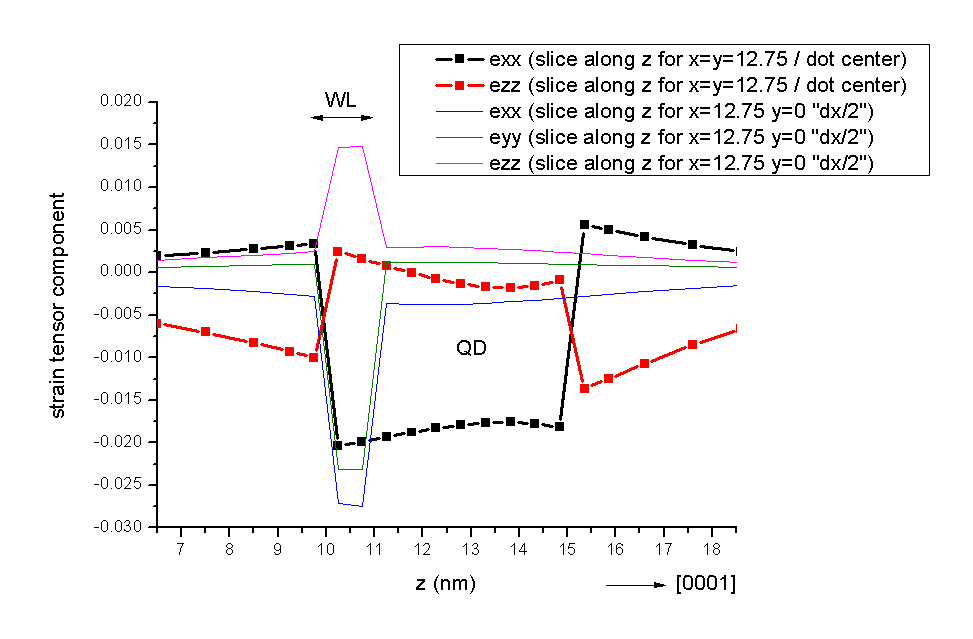The following figures show the strain tensor components along the [10-10] direction (x direction) for a line through the bottom of the quantum dot and for a line through the wetting layer.
These figures are related to Fig. 2 (b) in the cited paper of Andreev/O'Reilly.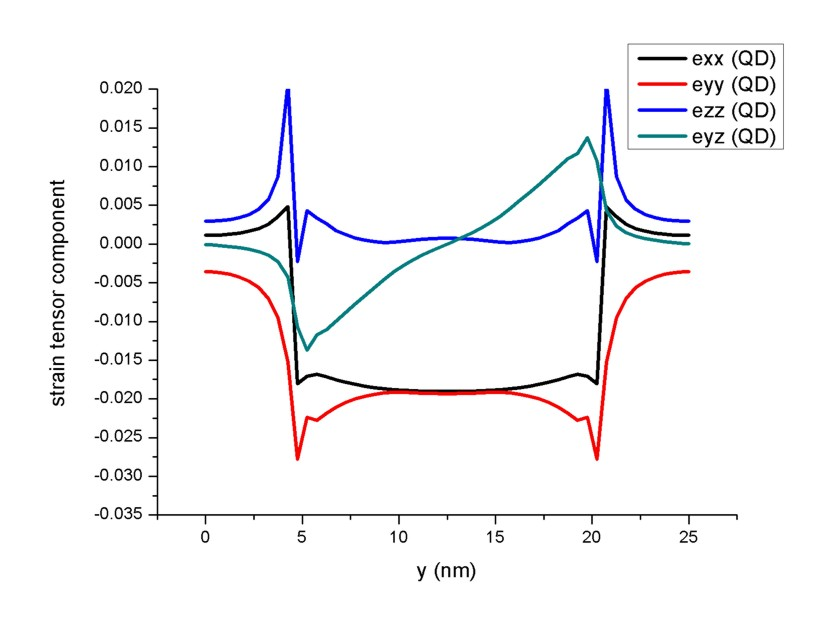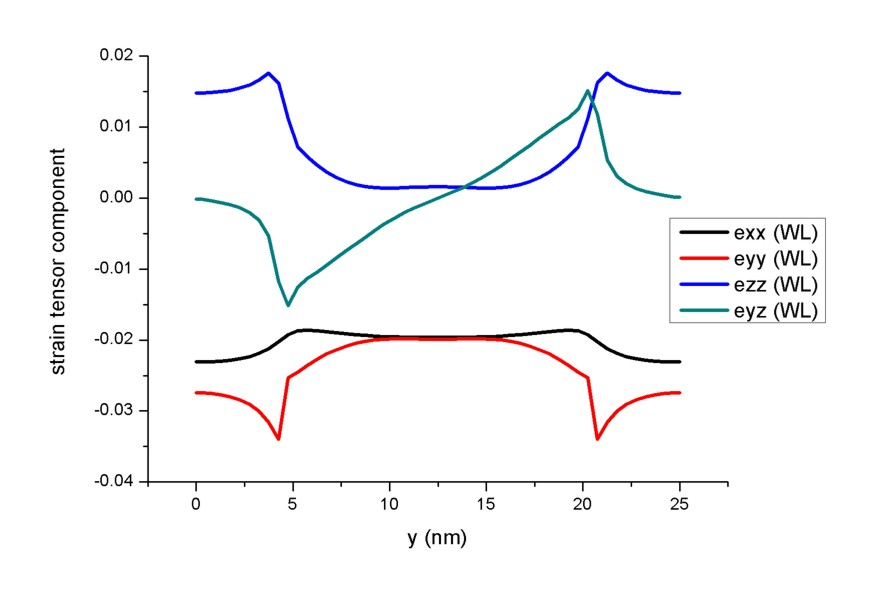The strain induced piezoelectric fields and the pyroelectric fields lead to the following electrostatic potential.
The figures of the potential are related to Fig. 4 in the cited paper of Andreev/O'Reilly.
In the left figure one can clearly see that the electrostatic potential has its maximum at the top of the QD and its minimum in the wetting layer area just below the QD.
The right figure shows a cut of the electrostatic potential through the wetting layer plane.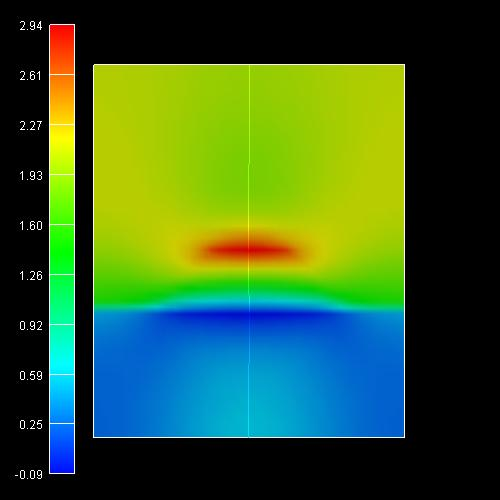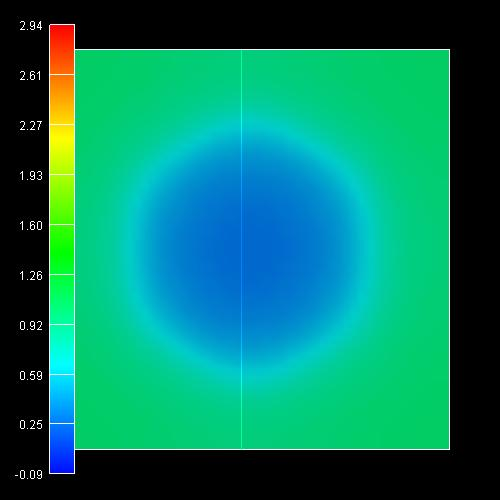The conduction (left) and valence band edges (right) are shown in the following figures.
One can clearly see that the conduction band minimum is located in the top of the quantum dot whereas the maximum for the valence band is located inside the wetting layer (WL) / bottom of the quantum dot. Thus one expects the electrons (located in the top area of the QD) to be spatially separated from the holes (located in the WL/bottom of the QD).
The energy scale is in units of [eV].
The figures of the conduction and valence band edges are related to Figs. 5 and 6 in the cited paper of Andreev/O'Reilly.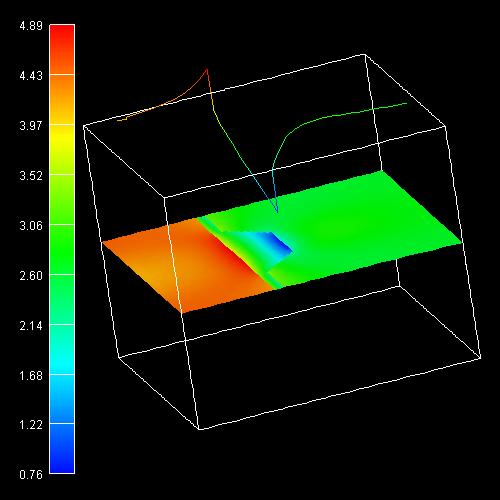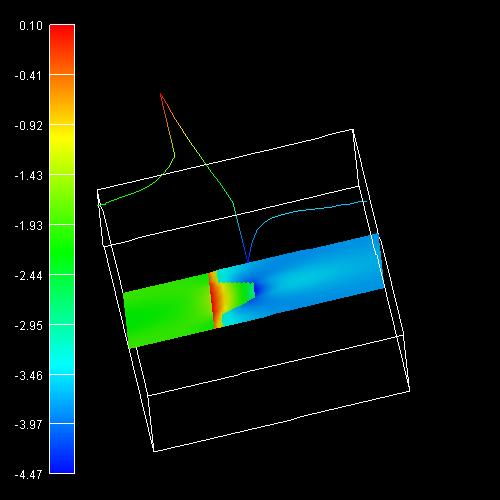The electron states are located near the top of the quantum dot where the conduction band has a minimum.
The following figure shows the electron ground state.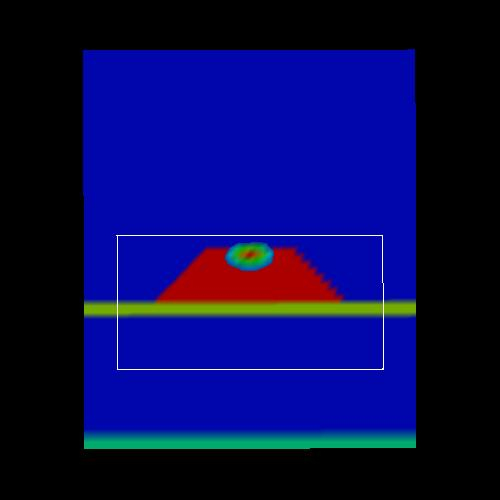The following figures show the six lowest electron states of the quantum dot. The 2nd and 3rd eigenstates are degenerate, as well as the 4th, 5th and 6th.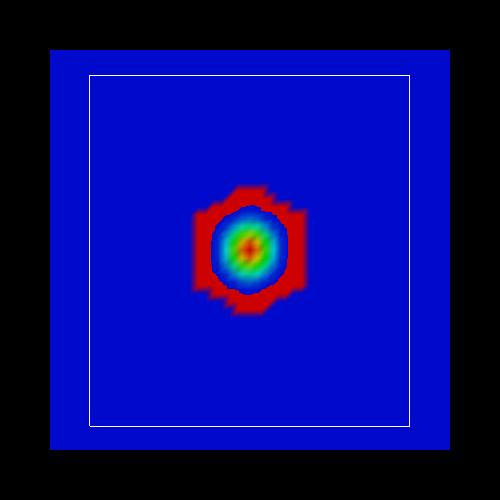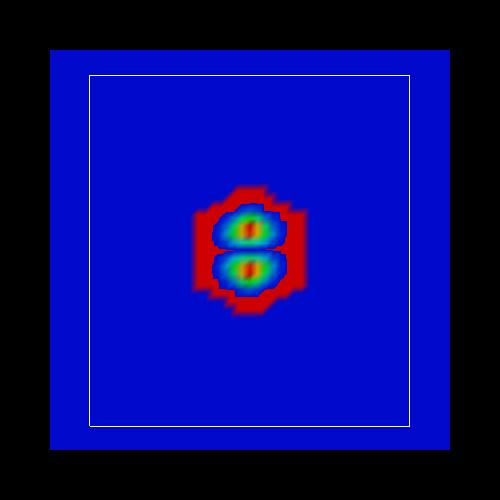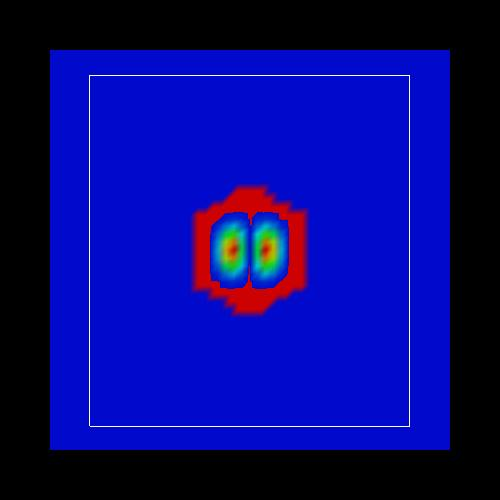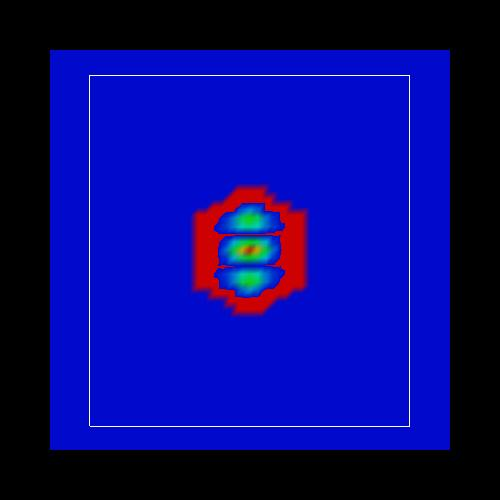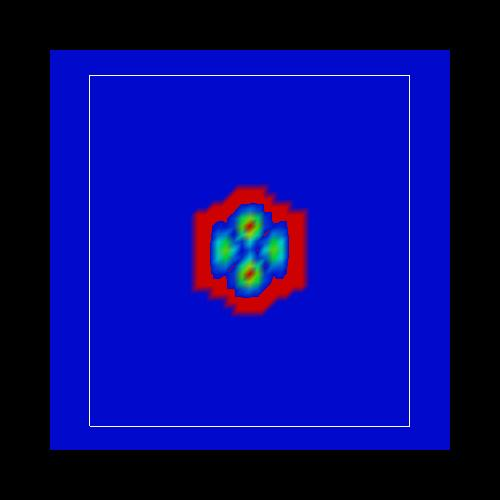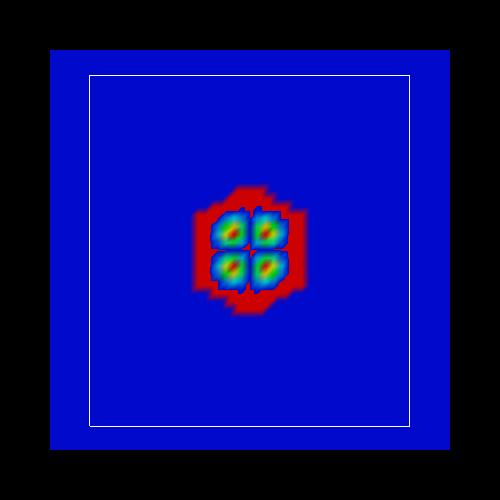The figures of the wave functions (psi²) are related to Fig. 7 in the cited paper of Andreev/O'Reilly.